Select Page

# 12 Science Maths CBSE Differential Equations Answers for MCQ in English

12 Science Maths CBSE Differential Equations Answers for MCQ in English to enable students to get Answers in a narrative video format for the specific question.

Expert Teacher provides 12 Science Maths CBSE Differential Equations Answers for MCQ through Video Answers in English language. This video solution will be useful for students to understand how to write an answer in exam in order to score more marks. This teacher uses a narrative style for a question from Differential Equations not only to explain the proper method of answering question, but deriving right answer too.

Please find the question below and view the Answer in a narrative video format.

Question:

## Similar Questions from CBSE, 12th Science, Maths, Differential Equations

Question 1 : Solve the differential equation: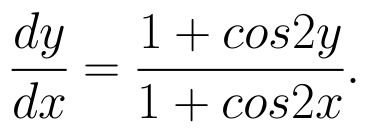(View Answer Video)

Question 2 : Solve the differential equation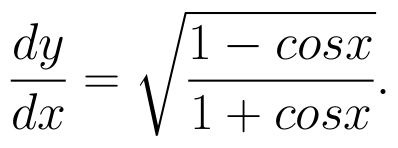(View Answer Video)

Question 3 : Find the particular solution of the differential equation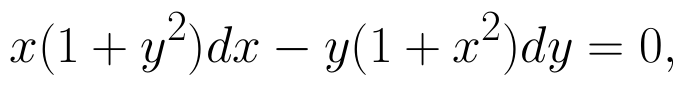given that y = 1 when x = 0.  (View Answer Video)

Question 4 : Write the differential equation formed from the equation y = mx + c, where m and c are arbitrary constants.

Question 5 : If y(x) is a solution of the differential equation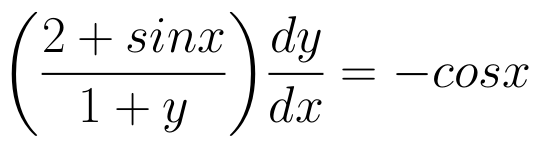and y(0) = 1, then find the value of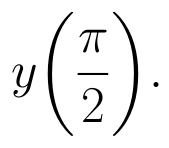(View Answer Video)

### Application of Derivatives

Question 1 : The rate of change of the area of a circle with respect to its radius r at r=6cm is: (View Answer Video)

Question 2 : If the radius of a sphere is measured as 9 m with an error of 0.03 m, then find the approximate error in calculating its surface area. (View Answer Video)

Question 3 : The slope of the normal to the curve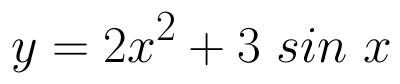at x = 0 is: (View Answer Video)

Question 4 : Find the equation of the normal to a curve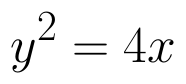which passes through the point (1,2). (View Answer Video)

Question 5 : Find two positive numbers x  and y such that x+y=60 and is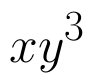maximum. (View Answer Video)

### Inverse Trigonometric Functions

Question 1 : Solve for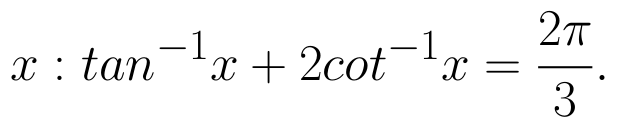(View Answer Video)

Question 2 : If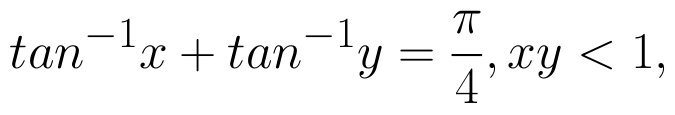then write the value of x + y + xy. (View Answer Video)

Question 3 : Solve for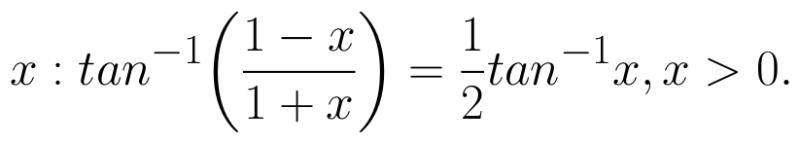(View Answer Video)

Question 4 : Find the greatest and least values of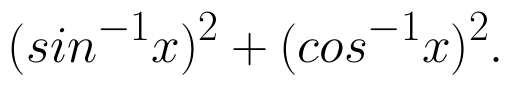(View Answer Video)

Question 5 : Write the value of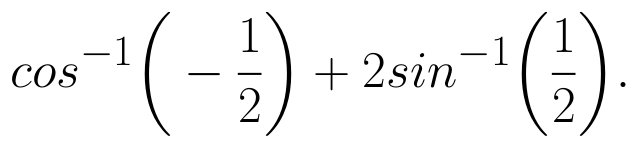(View Answer Video)

### Continuity and Differentiability

Question 1 : Differentiate the function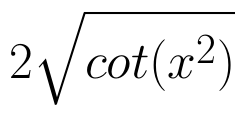with respect to x. (View Answer Video)

Question 2 : Differentiate the function w.r.t.x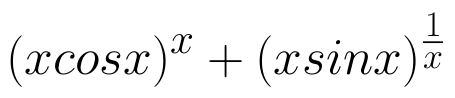. (View Answer Video)

Question 3 : Differentiate the function w.r.t.x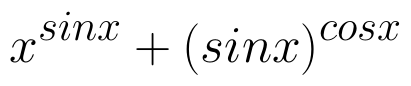. (View Answer Video)

Question 4 : If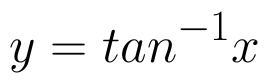, find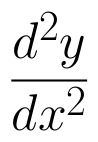in terms of y alone. (View Answer Video)

Question 5 : Differentiate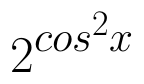w.r.t.x. (View Answer Video)